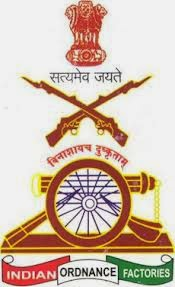# Indian Ordinance Factory Non Technical Online Test

80 Questions | Attempts: 156
ShareSettings• 1.
Currently Which one of the following is a Governer of Hariyana
• A.

Manohar lal Khattar

• B.

Kaptan singh Solanki

• C.

Kalyan singh

• D.

Jaswant singh

• 2.
What will be the last digit of the number obtained by multying the numbers 31x32x33x34x35x36x37
• A.

1

• B.

3

• C.

5

• D.

0

• 3.
The sum of the digits of a two-digit number is 10, while when the digits are reversed the number decrease by 54. find the changed number.
• A.

28

• B.

19

• C.

37

• D.

46

• 4.
What will be the L.C.M. of 5/2, 8/9, 11/14 .
• A.

280

• B.

360

• C.

420

• D.

440

• 5.
What will be HCF of the Polynomials  and
• A.

(x-2)

• B.

(x-1)

• C.

(x-3)

• D.

(x-5)

• 6.
If 146! Is divisible by 5n , then maximum value of n will be
• A.

34

• B.

35

• C.

36

• D.

37

• 7.
The sum of two numbers is 15 and their geometric mean is 20% lower than their arithmetic mean. Find the Numbers
• A.

11, 4

• B.

12, 3

• C.

13, 2

• D.

10, 5

• 8.
Which one of the following country was the organiser of FIFA-2014 World cup
• A.

Germany

• B.

Argentina

• C.

Brazil

• D.

Columbia

• 9.
Which of the following is a writer of the book "The Accidental Prime Minister -the making and unmaking of manmohan singh"
• A.

Sanjay Baru

• B.

P C Parekh

• C.

General VK Singh

• D.

S Y Kuraishi

• 10.
Currently which one of the chief of the indian army
• A.

Dalbir singh suhag

• B.

D K Joshi

• C.

Vikram singh

• D.

VK singh

• 11.
Which one of the following country which were selected for first Foreign visit of prime minister of india shri Narendra modi
• A.

Bhutan

• B.

Nepal

• C.

• D.

Japan

• 12.
Currently who is the main judge of supreme court
• A.

R M Lodha

• B.

V S Sampat

• C.

Ved Prakash

• D.

S K Roy

• 13.
What will be the reminder of 15X17X19 when divided by 7
• A.

1

• B.

3

• C.

5

• D.

7

• 14.
What will be the greatest number less than 10000, which is divisible by 48, 60, 64
• A.

9600

• B.

8500

• C.

7600

• D.

9800

• 15.
What will be the least comman multiple of 11 which when divided by 8, 9, 12, 14 leaves 4 as remainder in each case
• A.

1014

• B.

1037

• C.

1090

• D.

1012

• 16.
The LCM of two numbers is 12 times their HCF. The sum of HCF and LCM is 403. if one of the number is 93 what will be the other
• A.

134

• B.

124

• C.

128

• D.

126

• 17.
The speed of the train in going from Nagpur to allahabad is 100 km/hr while when coming from allahabad to nagpur, its speed is 150 km/hr What will be the average speed During the Journey
• A.

125 km/hr

• B.

120 km/hr

• C.

75 km/hr

• D.

135 km/hr

• 18.
The age of Mithali and pooja is in the ratio 2 : 6 After 5 years, the ratio of their ages will become 6 : 8 , Find the average of their ages after 10 years
• A.

12

• B.

13

• C.

17

• D.

24

• 19.
What will be Average of the First 97 Natural numbers
• A.

47

• B.

37

• C.

49

• D.

48

• 20.
The average of 5 Consecutive number is n. If the next two numbers are also included, the average will
• A.

Increase by 1

• B.

Increase by 2

• C.

Increase by 3

• D.

Remain same

• 21.
The ratio between two numbers is 3 : 4 and their LCM is 180. What will be the first number
• A.

60

• B.

45

• C.

15

• D.

20

• 22.
A mixture contains milk and water in the ratio 5 : 1 on adding 5 litres of water, the ratio of milk to water becomes 5 : 2, the quantity of milk in the mixture is
• A.

16 litres

• B.

25 litres

• C.

32.5 litres

• D.

22.75 litres

• 23.
If A : B = 7 : 5 and B : C = 9 : 11 then A : B : C is
• A.

55 : 45 : 63

• B.

63 : 45 : 55

• C.

45 : 63 : 55

• D.

45 : 65 : 60

• 24.
The number that must be added to each of numbers 8, 21, 13 and 31 to make the ratio of first two numbers equal to the ratio of last two number
• A.

5

• B.

7

• C.

9

• D.

1

• 25.
A number is mistakenly divided by 5 instead of being multiplied by 5. find the percentage change in the result due to this mistake
• A.

96%

• B.

95%

• C.

98%

• D.

94%

## Related TopicsBack to top
×

Wait!
Here's an interesting quiz for you.